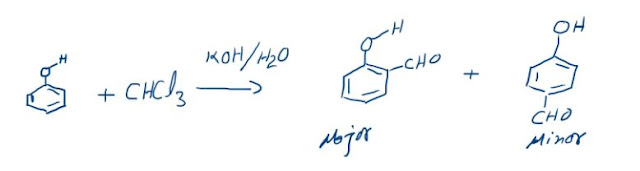### Photoelectric Effect

The emission of electrons from a metal surface on the incidence of electromagnetic radiations is called “Photoelectric Effect”.
The basic principle behind this effect is that the radiations transfer the energy to electrons inside the metal and if this energy is sufficient then the electrons are ejected from the surface. According to Maxwell’s Theory the ejection of electrons should depend on intensity of radiation that is if electrons are not being ejected, then on increasing the intensity they can be ejected and so on. The following observations are made: i.e. the energy of the electrons should have been proportional to the intensity of the light, not the frequency.
1. No electron is ejected if the incident frequency, ν, is less than certain minimum value ν0.
2. When ν < ν0 then even on increasing the intensity here is no ejection of electrons.
3. When ν > ν0, howsoever low the intensity is, ejection is observed.
4. When ν > ν0, on increasing the intensity the number of photoelectrons keep on increasing.
These findings again contradict Maxwell’s theory.

## Explanation of Photoelectric Effect:

As one electron absorbs one quantum that is why if the energy of one quantum is insufficient for the ejection of electrons then there is no use of increasing the number by increasing the intensity. To make ejection possible the energy per Quantum should be increased by increasing frequency. And for every metal there is a minimum value of frequency at which ejection starts which is called “Threshold Frequency” represented by “ν0”.
So the following observations were made:
1. When ν < ν0 then energy of one quantum is insufficient, ejection does not take place
2. When ν = ν0 then ejection just takes place and electrons don’t have any kinetic energy.
3. When ν > ν0 then every ejected electron possesses kinetic energy that is the excess energy of a quantum gets converted into kinetic energy of the electron. The Threshold energy required for emission is called “Work Function” (f) that is “h ν0”.
Thus by energy balance:
Energy of one quantum = Threshold Energy + Kinetic Energy
h ν = h ν0 + (1/2)mev2
4. When ν > ν0, then on increasing the intensity the number of quanta incident increases thereby increasing the number of photoelectrons ejected.
The Kinetic energy of electron varies linearly with the incident frequency as shown in the curve. Einstein gave this equation of photoelectric effect and he concluded that Light has particle like properties as well.
We can conclude that according to Maxwell, Light is a wave but according to modern theory it may also have particle like properties, as it contains photons each with discrete amount of energy. Therefore, Light has both the properties: Wave and Particle.

### The Reimer-Tiemann reaction: Reaction and its Mechanism

Reimer-Tiemann Reaction Phenols on reaction with chloroform in the presence of sodium hydroxide (or potassium hydroxide) solution give hydro...